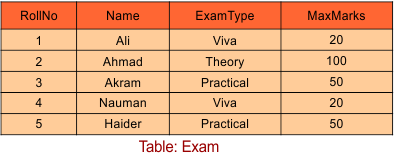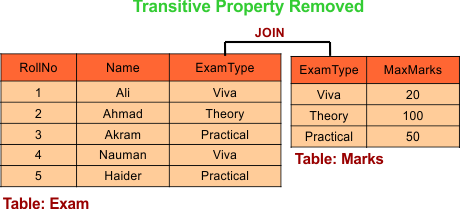Select Page

# Third Normal Form (3NF)

A table will be in the third normal form (3NF) when,

1. It is in the Second Normal form.

2. And, it does not have Transitive Dependency.

According to transitive property A determines B and B determine C. So, “A” can determines C through B, but not directly.

Let explain with Relational table

In the following table Roll_No is the candidate key which determines ExamType and ExamType determines the MaxMarks. So the following table holds transition property. That’s why it in not in 3NF.## Removal of Transitive Property

Transitive property can be removed by dividing the table into its parts.Let explain with Functional dependency example

## Conditions for Questions of 3NF

Any functional dependencies (FD) in relation will be 3NF, if it fulfill the following condition

L.H.S of FD should be a candidate or super key

OR

R.H.S of FD is a prime attribute.

## Explanation of 3NF with FD Rules

### Example 01:

Question: Consider a relation R =(ABCD) and  FD= (AB→ C, C→ D). Check Relation is in 3NF or Not?

Solution:

• CK ={ AB}
• Prime attribute ={ A, B}
• Non-prime Attribute= {C, D}

According to first FD (AB→ C) .The L.H.S of FD contains candidate key so, it is valid for 3rd NF.

According to Second FD (C→ D). The L.H.S of FD is not a candidate key or supper key And R.H.S is also a non-prime attribute. As both conditions false, So this FD is not valid for 3rd NF.

Conclusion: As above all FD’s of a relation are not valid, So relation is not in 3NF.

### Example 02:

Question: Consider a relation R =(ABCD) and  FD= (AB→CD, D→A). Check Relation is in 3NF or Not?

Solution:

• First find Candidate key (C.K) = {AB, DB}
• Second Find Prime attributes = {A, B, D}
• Third Find Non-Prime attributes = {C}

Now check for 3NF

• For first FD in Relation = AB→CD (As L.H.S contains the Candidate key so this FD is suitable for 3NF).
• For Second FD in Relation = D→A (As L.H.S does not contains the candidate or super key but R.H.S contains the prime attribute so this FD is suitable for 3NF.)

Result: As all FD’s of Relation fulfill the conditions of 3NF so this relation is in 3NF

Help Other’s By Sharing…